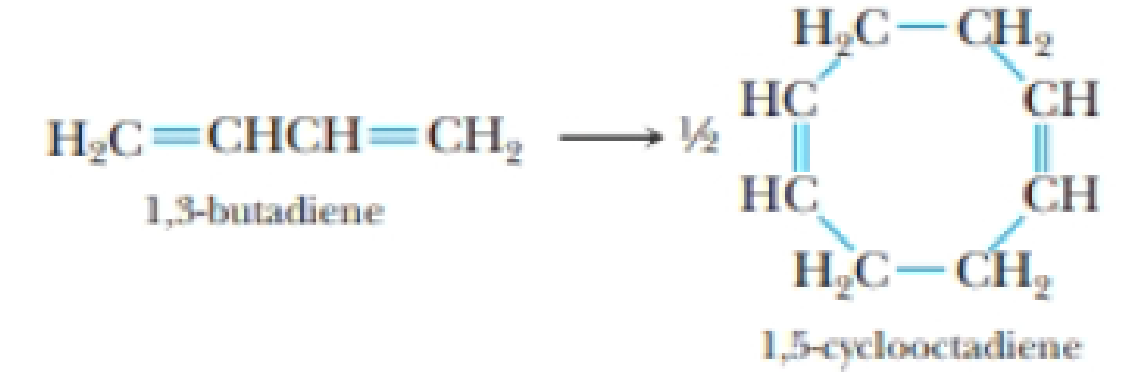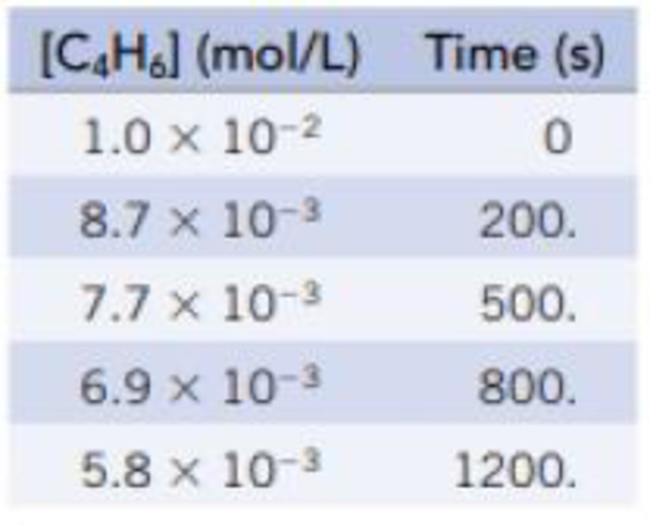Chapter 14, Problem 36PS

Chapter
Section
Textbook Problem

Butadiene, C4H6(g), dimerizes when heated, forming 1,5-cydooctadiene, C8H12. The data in the table were collected.(a) Use a graphical method to verify that this is a second-order reaction. (b) Calculate the rate constant for the reaction.

(a)

Interpretation Introduction

Interpretation:

The order for the given reaction should be determined using the given set of data.

Concept introduction:

Rate law: It is generally the rate equation that consists of the reaction rate with the concentration or the pressures of the reactants and constant parameters.

Rate constant: The rate constant for a chemical reaction is the proportionality term in the chemical reaction rate law which gives the relationship between the rate and the concentration of the reactant present in the chemical reaction.

Rate order: The order of each reactant in a reaction is represented by the exponential term of the respective reactant present in the rate law and the overall order of the reaction is the sum of all the exponents of all reactants present in the chemical reaction.  The order of the reaction is directly proportional to the concentration of the reactants.

Explanation

For the given reaction 1concentrationvstime are tabulated as follows by using the given set of data.

Figure 1

The corresponding graph for the above table is as follows,

(b)

Interpretation Introduction

Interpretation:

The rate constant for the given reaction should be determined using the given set of data.

Concept introduction:

Rate law: It is generally the rate equation that consists of the reaction rate with the concentration or the pressures of the reactants and constant parameters.

Rate constant: The rate constant for a chemical reaction is the proportionality term in the chemical reaction rate law which gives the relationship between the rate and the concentration of the reactant present in the chemical reaction.

Rate order: The order of each reactant in a reaction is represented by the exponential term of the respective reactant present in the rate law and the overall order of the reaction is the sum of all the exponents of all reactants present in the chemical reaction.  The order of the reaction is directly proportional to the concentration of the reactants.

Still sussing out bartleby?

Check out a sample textbook solution.

See a sample solution

The Solution to Your Study Problems

Bartleby provides explanations to thousands of textbook problems written by our experts, many with advanced degrees!

Get Started